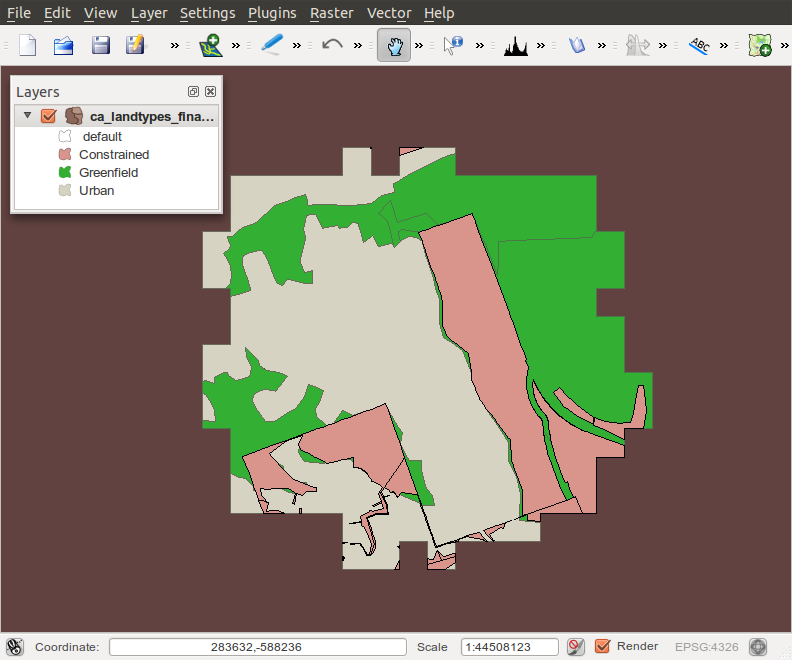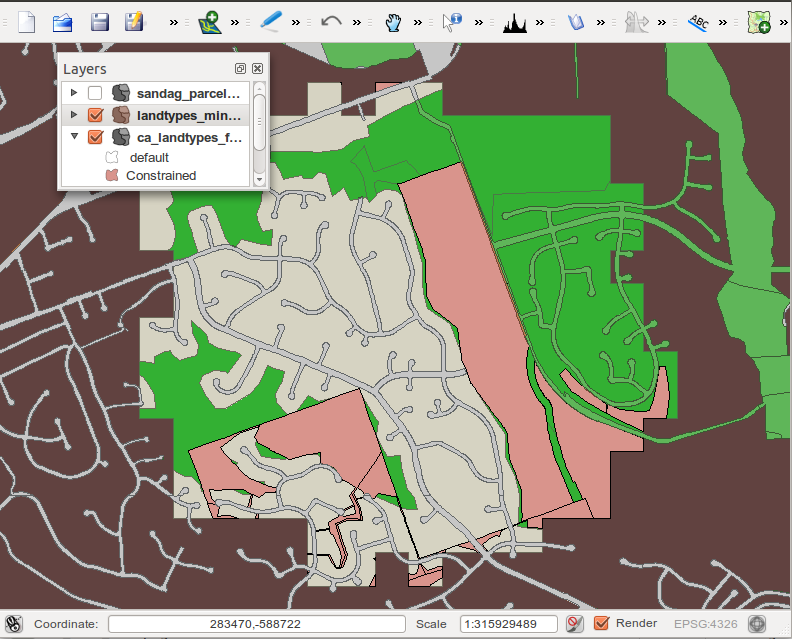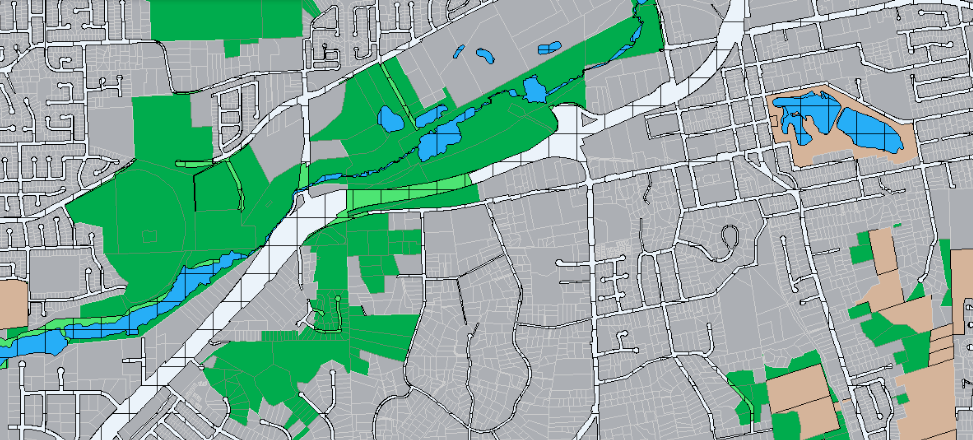## Extract and Sum Classified Areas by Grid CellGiven a set of classified polygons, in this example classified into three categories by name, apply a reference grid, and sum the areas for each classification into float fields in a destination table. This code contains test harness pieces in it also, so that a smaller example can be executed and verified, before applying the process to large sets.

```drop table if exists ca_lt1 cascade; --test_geom_out;
create table ca_lt1 --test_geom_out
as    select
g.gridcode,
g.the_geom,
SUM(d.acres_urban) as acres_urban,
SUM(d2.acres_constrained) as acres_constrained,
SUM(d3.acres_greenfield) as acres_greenfield
FROM
grid150m_uniq g
--test_grid g
LEFT JOIN
( select
sum(st_area( st_intersection( land.wkb_geometry, g2.the_geom))) as acres_urban,
g2.gridcode
from
grid150m_uniq g2, --test_geom land
ca_landtypes_df land
where st_intersects( land.wkb_geometry, g2.the_geom) AND
land.landtype = 'Urban'
AND st_intersects( st_geomfromEWKT('SRID=3310;POLYGON((-147472.090  71129.874, -113634.730  71129.874,   -113634.730  50253.940,   -147472.090 50253.940,  -147472.090 71129.874))'), g2.the_geom)
group by g2.gridcode
) d
ON ( g.gridcode = d.gridcode )

LEFT JOIN
( select
sum(st_area( st_intersection( land.wkb_geometry, g2.the_geom))) as acres_constrained,
g2.gridcode
from
grid150m_uniq g2, --test_geom land
ca_landtypes_df land
where st_intersects( land.wkb_geometry, g2.the_geom) AND
land.landtype = 'Constrained'
AND st_intersects( st_geomfromEWKT('SRID=3310;POLYGON((-147472.090  71129.874, -113634.730  71129.874,   -113634.730  50253.940,   -147472.090 50253.940,  -147472.090 71129.874))'), g2.the_geom)
group by g2.gridcode
) d2
ON ( g.gridcode = d2.gridcode )

LEFT JOIN
( select
sum(st_area( st_intersection( land.wkb_geometry, g2.the_geom))) as acres_greenfield,
g2.gridcode
from
grid150m_uniq g2, --test_geom land
ca_landtypes_df land
where st_intersects( land.wkb_geometry, g2.the_geom) AND
land.landtype = 'Greenfield'
AND st_intersects( st_geomfromEWKT('SRID=3310;POLYGON((-147472.090  71129.874, -113634.730  71129.874,   -113634.730  50253.940,   -147472.090 50253.940,  -147472.090 71129.874))'), g2.the_geom)
group by g2.gridcode
) d3
ON ( g.gridcode = d3.gridcode )

WHERE st_intersects( st_geomfromtext('SRID=3310;POLYGON((-147472.090  71129.874, -113634.730  71129.874,   -113634.730  50253.940,   -147472.090 50253.940,  -147472.090 71129.874))'), g.the_geom)

GROUP BY
g.gridcode,
g.the_geom;

```